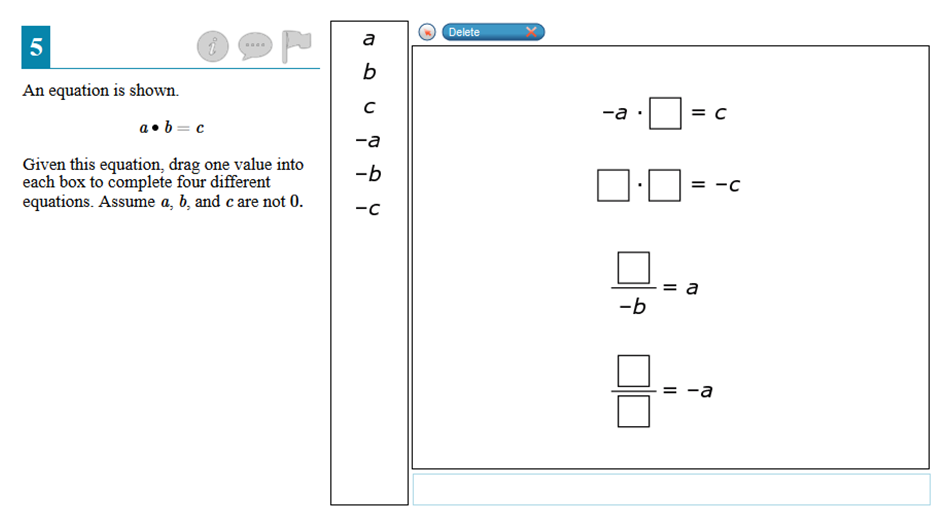Home > Grade 7 > Equivalent Equations

# Equivalent Equations### Hint

How do negatives affect multiplication and division?
What is missing from each of the equations?